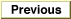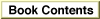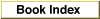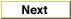# Legacy DocumentImportant: The information in this document is obsolete and should not be used for new development.

Inside Macintosh: QuickDraw GX Environment and Utilities /
Chapter 8 - QuickDraw GX Mathematics / QuickDraw GX Mathematics Reference
Mathematical Functions / Fixed-Point Operations

### FractSineCosine

You can use the `FractSineCosine` function to obtain both the sine and cosine of an angle measured in degrees.

```fract FractSineCosine (Fixed degrees, fract *cosine);
```
`degrees`
The angle in degrees for which the cosine and sine are required.
`cosine`
A pointer to the location where the cosine of the angle is required.
function result
The sine of the angle specified.
##### DESCRIPTION
Given the `degrees` parameter in degrees, the `FractSineCosine` function returns the sine as the function result and the cosine in the `cosine` parameter. Values for the `degrees` parameter are specified in degrees, not radians. The range of the angle is -32,768 to +32,769.999 degrees.